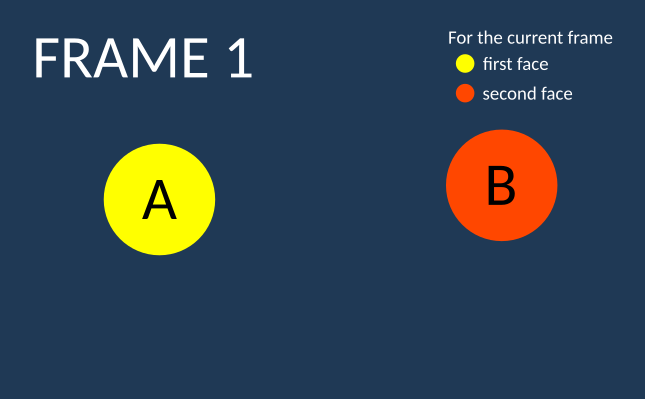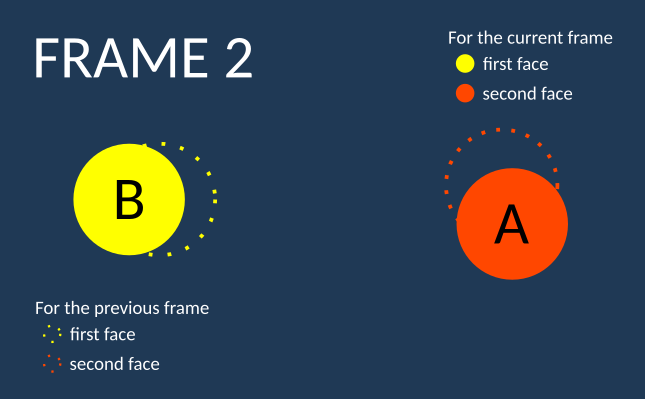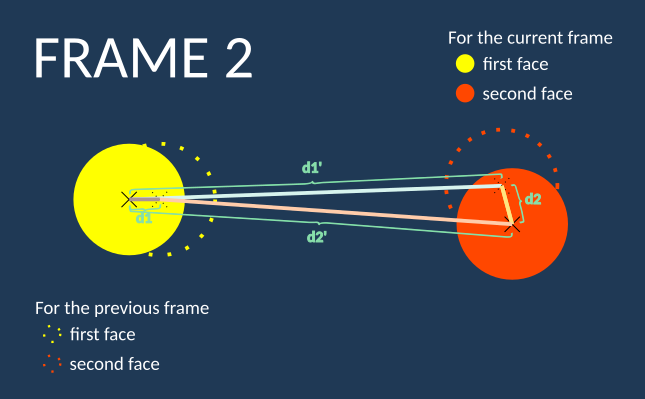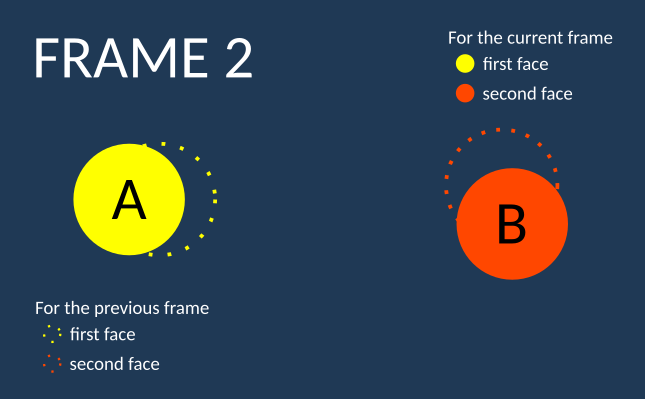Did you know that you can navigate the posts by swiping left and right?

# Face detector and the Hungarian method

#### 31 Dec 2017 . category: tech . Comments #gnome #cheese #opencv #dlib #computer vision #cv

It has been long time I don’t write here, but I was bussy with many things I try to do at the same time. What is interesting is that it seems that I will be able to deal with it. But something that I didn’t comment publicly is that as part of my dissertation project or thesis project I proposed to implement a multiple face detector and tracker to use it to apply Purikura-like effects in Cheese. I have done some research during the last academic semester about how to implement this in a course called Thesis Project I, where students should focus only in researching about the topic they will treat in their project. Now that the course has finished I have time to try things that I researched on, and I am very happy because I could solve a problem when I tried to add support for multiple faces in gstfaceoverlay during the first time I wanted to implement these kind of effects.

The problem I had which was described in an old post was that the OpenCV face detector does not have sense of order. If there are 2 people in the scene, the first person can be tagged with A and the second with B. In the next frame you would expect that the first person would be tagged with A and the second with B as it was before, but as I said the OpenCV face detector does not have sense of order. I have solved this problem and it was using the hungarian method. The idea I have applied is very simple.

Note: dlib’s and OpenCV’s face detector gives as the bounding boxes for each faces. When I talk about the position of the detected faces I refer to the centroid of that bounding box.

So let’s suppose we are in the first frame. There are two people in there: the first one represented by the yellow circle and the second one represented by the red circle. Our face detector tells us that the first person is A and the second person is B.Then in the second frame, people have moved and thus their faces too, so the faces changed their positions. However when the face detector is run for this frame, our face detector tells us that the first person is B and the second person is B. That’s not how it should supposedly be. Because what we want is that as in the first frame the first person should be tagged again with A and the second one with B.How do we solve this problem? At least the first person had teletransportation powers, it is expected that the position of his/her face in the next frame will be pretty close to the previous position. The same for the second person, his/her face shouldn’t have gone pretty far. We need to find some way to match for each face in the current detected frame, which face it belongs to against the previous frame. So the task is simple. For the first detected person, I calculate its (Euclidean) distance to the previous detected faces… and for the second person, I do the same. The next task is to see what are the shorter distances. Here is when the Hungarian method comes.The Hungarian method is an algorithm developed for solving assignment problems. From Wikipedia we have a simple problem that they use to explain how it can be used. I directly cite them:

In this simple example there are three workers: Armond, Francine, and Herbert. One of them has to clean the bathroom, another sweep the floors and the third washes the windows, but they each demand different pay for the various tasks. The problem is to find the lowest-cost way to assign the jobs. The problem can be represented in a matrix of the costs of the workers doing the jobs. For example:

Clean bathroom Sweep floors Wash windows
Armond \$2 \$3 \$3
Francine \$3 \$2 \$3
Herbert \$3 \$3 \$2

The Hungarian method, when applied to the above table, would give the minimum cost: this is \$6, achieved by having Armond clean the bathroom, Francine sweep the floors, and Herbert wash the windows.

With the data of the distances calculated prevoously for the previous and current frame, I build my matrix of distances (or costs) as following:

First person in frame 1 Second person in frame 1
First person in frame 2 d1 d1’
Second person in frame 2 d2’ d2

The Hungarian method would give as that the first person in frame 2 should be matched to the first person in frame 1 and that the second person in the frame 2 should be matched to the second person in frame 2. Easily explaining because d1 is less than d1’ and d2 is less than d2’. So we can reorder our tags as A and B (instead of B and A). So we would tag the faces as in the figure below:You can see a demonstration with these videos. This first is using just a face detector. You can see how fast the red and blue boxes switch fastly in the subsuquent frames. The idea was to fix this:

Then in the video below you can see how the blue and green boxes are stick to the faces they belong to:

And basically that’s it for the purpose of this post. More complex things needs to be done to have a better tracker like probably applying the Kalman Filter and some other methods to still capture a face when even the face detector fails. If you prefer code, you have some code here. I have used as a face detector dlib, OpenCV for capturing from the webcam and an algorithm I found googling for the Hungarian method, because dlib’s one was not enough if I have an unbalanced matrix (when the number of rows differs from columns). The code I show has some things that are not really necessary and could have been simplied more, but I tell you that I have removed some lines of code there, because it had more because I was implementing tracking using the Kalman Filter. Whatever question or suggestion just left a comment :)

``````/*
* Copyright (C) 2017 Fabian Orccon <cfoch.fabian@gmail.com>
*
* This program is free software; you can redistribute it and/or
* modify it under the terms of the GNU General Public License
* as published by the Free Software Foundation; either version 2
* of the License, or (at your option) any later version.
*
* This program is distributed in the hope that it will be useful,
* but WITHOUT ANY WARRANTY; without even the implied warranty of
* MERCHANTABILITY or FITNESS FOR A PARTICULAR PURPOSE.  See the
* GNU General Public License for more details.
*
* You should have received a copy of the GNU General Public License
* along with this program; if not, write to the Free Software
* Foundation, Inc., 51 Franklin Street, Fifth Floor, Boston, MA  02110-1301, USA.
*/

#include <cstdio>
#include <vector>
#include <valarray>
#include <algorithm>

#include <dlib/image_processing/frontal_face_detector.h>
#include <dlib/image_io.h>
#include <dlib/gui_widgets.h>
#include <dlib/opencv.h>
#include "opencv2/opencv.hpp"

#include "Hungarian.h"

#define FACE_ASPECT_RATIO     1.25

using namespace cv;
using namespace std;
using namespace dlib;

static std::vector<Scalar> COLORS = {
Scalar(255, 0, 0), // RED
Scalar(0, 255, 0), // BLUE
Scalar(0, 0, 255), // GREEN
Scalar(255, 128, 0), // ORANGE
Scalar(255, 255, 0), // YELLOW
Scalar(0, 255, 255), // CYAN
Scalar(255, 0, 255), // FUCCSHIA
};

static Scalar
random_color(RNG &rng)
{
int icolor = (unsigned) rng;
return Scalar(icolor & 255, (icolor >> 8) & 255, (icolor >> 16) & 255);
}

static cv::Point
calculate_centroid(cv::Point & p1, cv::Point & p2)
{
return (p1 + p2) / 2;
}

static cv::Point
calculate_centroid(dlib::rectangle & rectangle)
{
cv::Point tl, br;
tl = cv::Point(rectangle.left(), rectangle.top());
br = cv::Point(rectangle.right(), rectangle.bottom());
return (tl + br) / 2;
}

class FaceTracker {
private:
cv::Point centroid;
bool have_measurement;
dlib::rectangle measurement_info;

public:
dlib::rectangle bounding_box;
Scalar color;

FaceTracker(dlib::rectangle &bounding_box)
{
this->bounding_box = bounding_box;
centroid = calculate_centroid(bounding_box);
}

cv::Point
get_centroid(void)
{
return centroid;
}

void
set_color(Scalar & color)
{
this->color = color;
}

void
set_have_measurement(bool value)
{
have_measurement = value;
}

void
set_measurement_info(dlib::rectangle measurement_info)
{
have_measurement = true;
this->measurement_info = measurement_info;
}

void
predict(void) {
Mat prediction, estimation;

cv::Point estimated_position;
int estimated_width;
bounding_box = measurement_info;
}
};

class MultiFaceTracker {
public:
std::vector<FaceTracker> trackers;

MultiFaceTracker()
{
}

MultiFaceTracker(std::vector<dlib::rectangle> &dets)
{
int i;
for (i = 0; i < dets.size(); i++) {
Scalar color = COLORS[i % COLORS.size()];
FaceTracker tracker = FaceTracker(dets[i]);
tracker.set_color(color);
trackers.push_back(tracker);
}
}

void
track(std::vector<dlib::rectangle> &dets)
{
int r, c, i;
std::vector<std::vector<double>> cost_matrix;
std::vector<int> assignment;

std::vector<cv::Point> cur_centroids;

//std::vector<cv::Point *> new_centers(real_centers.size(), NULL);
std::vector<dlib::rectangle> new_dets;
HungarianAlgorithm HungAlgo;

cout << "Calculate current centroids" << endl;
// Calculate current centroids.
for (i = 0; i < dets.size(); i++) {
cv::Point centroid = calculate_centroid(dets[i]);
cur_centroids.push_back(centroid);
}

cout << "Initialize cost matrix" << endl;
// Initialize cost matrix.
for (r = 0; r < trackers.size(); r++) {
std::vector<double> row;
for (c = 0; c < cur_centroids.size(); c++) {
float dist;
dist = cv::norm(cv::Mat(cur_centroids[c]),
cv::Mat(trackers[r].get_centroid()));
row.push_back(dist);
}
cost_matrix.push_back(row);
}

cout << "Solve the Hungarian assignment problem" << endl;
HungAlgo.Solve(cost_matrix, assignment);

cout << "assignment: ";
for (i = 0; i < trackers.size(); i++)
cout << assignment[i] << " ";
cout << endl;

cout << "Reorder faces" << endl;
// Reorder faces.
for (i = 0; i < trackers.size(); i++) {
if (assignment[i] == -1) {
cout << "A face was not detected" << endl;
trackers[i].set_have_measurement(false);
} else
trackers[i].set_measurement_info(dets[assignment[i]]);
trackers[i].predict();
}
cout << "Finish tracking for this frame" << endl;
}
};

int
main(int argc, const char ** argv)
{
VideoCapture cap;
const char * filename;
namedWindow("capture", WINDOW_AUTOSIZE);
frontal_face_detector detector;
std::vector<dlib::rectangle> prev_dets;
std::vector<cv::Point *> real_centers;
bool detected = false;
bool tracker_initialized = false;
int was_detected = false;
int frame_number = 1;
int frame_number_when_trackers_initialized = -1;
MultiFaceTracker multi_tracker;

cout << "naargs: " << argc << endl;

if (argc < 2) {
cerr << "This program receives a filename as an argument" << endl;
exit (1);
}

filename = argv;
cap.open(filename);

if (!cap.isOpened ()) {
cerr << "There was a problem opening '" << filename << "'" << endl;
exit (1);
}

detector = get_frontal_face_detector();
for (;;) {
Mat frame;
std::vector<cv::Point *> cur_centers;
std::vector<dlib::rectangle> dets;
int key_pressed;
int i;

cout << "Frame: " << i << endl;

cap >> frame;
break;

cv_image<bgr_pixel> dlib_image(frame);
dets = detector(dlib_image);

// Always try to detect faces.
detected = dets.size() > 0;
for (i = 0; i < dets.size(); i++) {
cv::Point tl, br;
cv::Point *centroid;
tl = cv::Point(dets[i].left(), dets[i].top());
br = cv::Point(dets[i].right(), dets[i].bottom());

//centroid = new cv::Point((tl + br) / 2);
//cur_centers.push_back(centroid);

// Initialize.
if (frame_number_when_trackers_initialized == -1) {
frame_number_when_trackers_initialized = frame_number;
multi_tracker = MultiFaceTracker(dets);
}
}

// Only do this for frames after the one in which the trackers were
// initialized.
if (frame_number_when_trackers_initialized > 0 &&
frame_number > frame_number_when_trackers_initialized) {
multi_tracker.track(dets);
for (i = 0; i < multi_tracker.trackers.size(); i++) {
cv::Point tl, br;
dlib::rectangle bounding_box = multi_tracker.trackers[i].bounding_box;
tl = cv::Point(bounding_box.left(), bounding_box.top());
br = cv::Point(bounding_box.right(), bounding_box.bottom());

cout << "Bounding box: " << i << endl;
cout << "top_left: " << tl << endl;
cout << "bottom_right: " << br << endl;

cv::rectangle(frame, tl, br, multi_tracker.trackers[i].color);
}
}

imshow ("capture", frame);

frame_number++;
// Change this to 100000000 to go frame by frame by pressing ENTER.
// ESC to quit.
key_pressed = waitKey (30);
if (key_pressed == 13)
continue;
else if (key_pressed == 27)
break;
}

return 0;
}
``````

And here it is a CMakeLists.txt file I used that may be useful if you want to compile that code.

``````cmake_minimum_required(VERSION 2.8.12)
project( KalmanHungarianTracking )